Two masses of 5kg and 10kg are connected to a pulley as shown. What will be the acceleration of the system (g = acceleration due to gravity)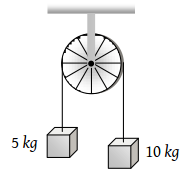(1) g

(2) $\frac{g}{2}$

(3) $\frac{g}{3}$

(4) $\frac{g}{4}$

Concept Questions :-

Application of laws
High Yielding Test Series + Question Bank - NEET 2020

Difficulty Level:

A block A of mass 7 kg is placed on a frictionless table. A thread tied to it passes over a frictionless pulley and carries a body B of mass 3 kg at the other end. The acceleration of the system is (given g = 10 ms–2)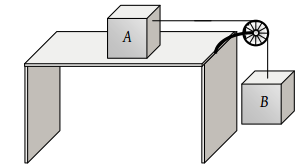(1) 100 ms–2

(2) 3 ms–2

(3) 10 ms–2

(4) 30 ms–2

Concept Questions :-

String constraint
High Yielding Test Series + Question Bank - NEET 2020

Difficulty Level:

Three blocks of masses 2 kg, 3 kg and 5 kg are connected to each other with light string and are then placed on a frictionless surface as shown in the figure. The system is pulled by a force F = 10 N, then tension T1 =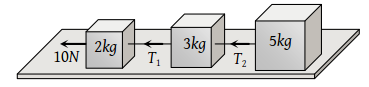(1) 1N

(2) 5 N

(3) 8 N

(4) 10 N

High Yielding Test Series + Question Bank - NEET 2020

Difficulty Level:

Two masses m1 and m2 are attached to a string which passes over a frictionless smooth pulley. When m1 = 10 kg, m2 = 6 kg, the acceleration of masses is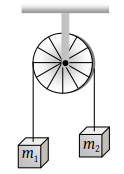(1) 20 m/s2

(2) 5 m/s2

(3) 2.5 m/s2

(4) 10 m/s2

Concept Questions :-

Application of laws
High Yielding Test Series + Question Bank - NEET 2020

Difficulty Level:

A body of weight 2kg is suspended as shown in the figure. The tension T1 in the horizontal string (in kg wt) is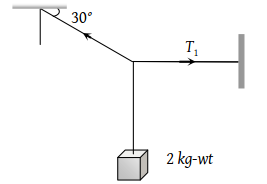(1) $2/\sqrt{3}$

(2) $\sqrt{3}/2$

(3) $2\sqrt{3}$

(4) 2

Concept Questions :-

Types of forces
High Yielding Test Series + Question Bank - NEET 2020

Difficulty Level:

One end of a massless rope, which passes over a massless and frictionless pulley P is tied to a hook C while the other end is free. Maximum tension that the rope can bear is 360 N. with what value of minimum safe acceleration (in ms–2) can a monkey of 60 kg move down on the rope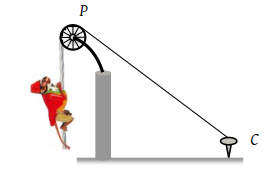(1) 16

(2) 6

(3) 4

(4) 8

Concept Questions :-

Application of laws
High Yielding Test Series + Question Bank - NEET 2020

Difficulty Level:

A light string passing over a smooth light pulley connects two blocks of masses m1 and m2 (vertically). If the acceleration of the system is g/8 then the ratio of the masses is

(1) 8 : 1

(2) 9 : 7

(3) 4 : 3

(4) 5 : 3

Concept Questions :-

Application of laws
High Yielding Test Series + Question Bank - NEET 2020

Difficulty Level:

Two masses m1 = 5 kg and m2 = 4.8 kg tied to a string are hanging over a light frictionless pulley. What is the acceleration of the masses when they are free to move (g = 9.8 m/s2)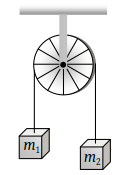(1) 0.2 m/s2

(2) 9.8 m/s2

(3) 5 m/s2

(4) 4.8 m/s2

Concept Questions :-

Application of laws
High Yielding Test Series + Question Bank - NEET 2020

Difficulty Level:

A block of mass 4 kg is suspended through two light spring balances A and B. Then A and B will read respectively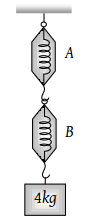(1) 4 kg and zero kg

(2) Zero kg and 4 kg

(3) 4 kg and 4 kg

(4) 2 kg and 2 kg

Concept Questions :-

Spring force
High Yielding Test Series + Question Bank - NEET 2020

Difficulty Level:

Two masses M and M/2 are joint together by means of a light inextensible string passes over a frictionless pulley as shown in figure. When bigger mass is released the small one will ascend with an acceleration of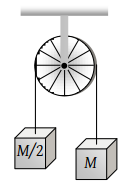(1) g/3

(2) 3g/2

(3) g/2

(4) g

Concept Questions :-

Application of laws